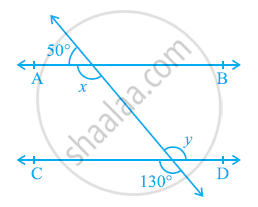Share

# In the given figure, find the values of x and y and then show that AB || CD. - CBSE Class 9 - Mathematics

ConceptLines Parallel to the Same Line

#### Question

In the given figure, find the values of x and y and then show that AB || CD.#### Solution

It can be observed that,

50º + x = 180º (Linear pair)

x = 130º        … (1)

Also, y = 130º (Vertically opposite angles)

As x and y are alternate interior angles for lines AB and CD and also measures of these angles are equal to each other, therefore, line AB || CD.

Is there an error in this question or solution?

#### APPEARS IN

NCERT Solution for Mathematics Class 9 (2018 to Current)
Chapter 6: Lines and Angles
Ex. 6.20 | Q: 1 | Page no. 103

#### Video TutorialsVIEW ALL 

Solution In the given figure, find the values of x and y and then show that AB || CD. Concept: Lines Parallel to the Same Line.
S